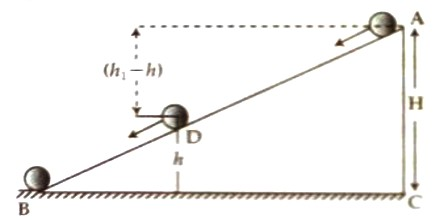# Verification of Galileo’s Law of a Falling body

Verification of Galileo’s Law of a Falling body

Theory: When a body is allowed to fall it falls downward at the action of gravity. Normally, a body falls from such a height which is very small compared to the radius of the earth. For this reason, it may be considered that the value of acceleration due to gravity acting on the falling body is constant. If there is no air resistance i.e., if the body falls freely, then these laws are applicable for that motion.

In case of a body falling freely from rest –

• In vacuum, all bodies fall with sane rapidity.
• The velocity which the body acquires at any time is proportional to that time.
• The distance that the body travels at a particular time is inversely proportional to the square of that time.

Apparatus:

1. An inclined smooth surface
2. A metre scale
3. A stop watch
4. A few marblesExperimental procedure: Place an inclined surface AB on the ground BC as shown in figure. Measure the height of point A from the ground by a metre scale. Now put some marks by a chalk on the inclined surface.

First, a marble is placard at point A and allowed to tall as soon as the stop watch starts and stops the watch when the ball reaches the point D. Now measure the distance between A and D with a metre scale and note down the time from the watch. Again, take the marble to point A and allow it to tall in similar way and measure time and distance of the inclined surface as soon as it touches the ground B. In this way measure the time and distance for a few points.

Repeat this experiment for other two marbles.

Repeat this experiment for other two marbles.

Graph of distance versus (time)2: Taking (time)2 X-axis and distance H along Y-axis draw a graph in a graph paper. The graph will be as shown in figure 2.Proof of the first law: If the surface is smooth and if there is no effective air resistance, then it will be seen that all the marbles will reach the ground or the point D at the same time. That means, if friction and air resistance are not effective, then all the marbles travel same distance at the same time. Hence, the first law of Galileo for the falling bodies is proved.

Proof of the second law: By measuring distances H1, H2 and H3 and corresponding times for travel t1, t2 and t3 for the marbles 1, 2 and 3 respective velocities v1, v2 and v3 is determined. It is seen that v1/t1 = v2/t2 = v3/t3 = Constant. That means v/t = constant or v ∞ t.

So, it is observed that velocity is proportional to time. Hence second law is verified.

Proof of the third law: From the graph 2, H1/t12, H2/t22 and H3/t32 for the three marbles are determined. It is seen that H1/t12 = H2/t22 = H3/t32 = constant.

That means, H/t2 = constant or, H ∞ t2

i.e., distance travelled at a particular time is proportional to the square of that time. So, third law is proved.

Precautions and discussions:

(1) Each marble is to be released from the same point of the vertex of the inclined plane.

(2) Care should be taken so that no resistance exists while the marble is ending.

(3) Distance and time should be measured correctly.

(4) Care should be taken so that in the experimental place there is no resistance of while the marble is descending.